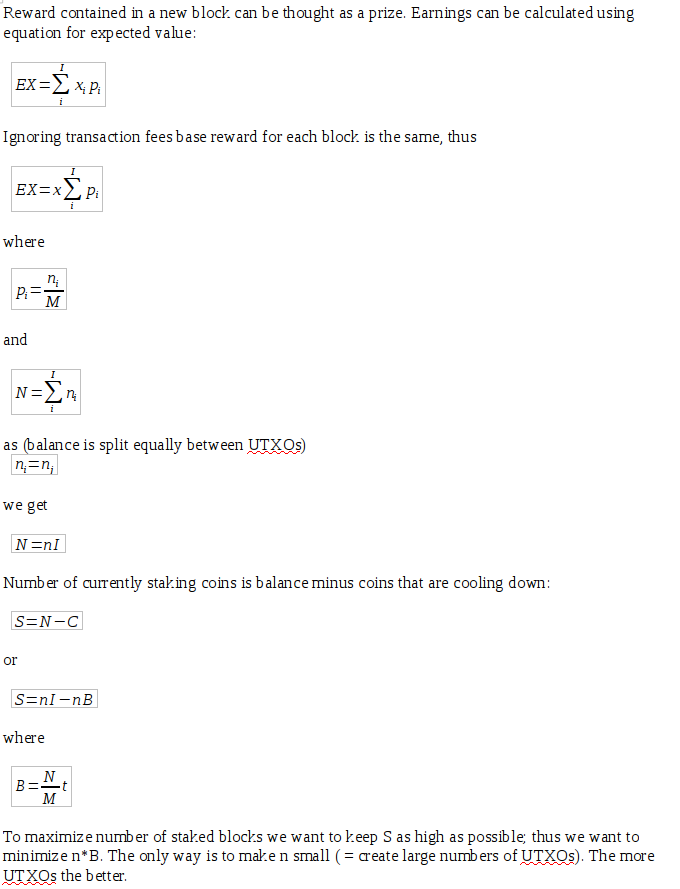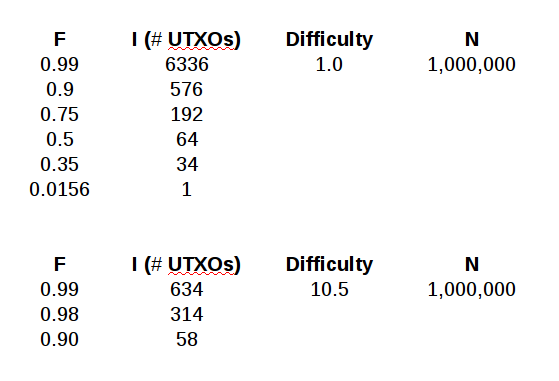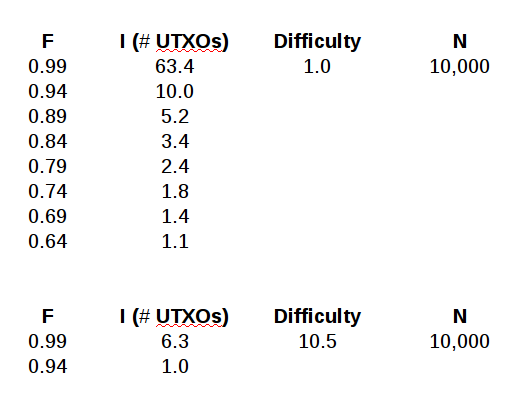# CBR – The Way to Split Coins (DRAFT)

in gridcoin •  last year  (edited)

Holding coins in Gridcoin blockchain allows to earn interest (~1.5% / year). To collect interest, you need to stake coins – come online. A large holder can come online for a few hours just once in a few months and earn whole accumulated interest. He could earn slightly more (due to compounding interest) if staking daily, but difference is negligible. This mechanism is causing low weight of the network that leads to several problems. To force higher network wait, Constant Block Reward (CBR) mechanism is researched. Each new block will reward staker with around 10 coins (exact number not decided yet). As coins need to mature for 16 hours to be able to stake, miner with just one UTXO will be able to stake no more often than just once within 16 hours (cooling down or maturing) period. Splitting balance between a number of UTXOs will allow, especially for whales, to maximize CRB rewards. Let’s assume you have 1 million coins and there are 10 million coins trying to stake a block. Out of each 640 blocks you can stake on average ~ 64 blocks. But with all coins in just one UTXO, after staking all of them would would have to mature for the next 640 blocks. As a result you would get only up to 1 / 64 of the possible reward.

The questions are: How to split coins? Does CBR mechanism impact small holders as much as large holders?

NOTE: An equation you will find below needs another pair of eyes to review and myself I have to re-check some steps. This is still a draft.

N – number of coins you hold
t – (time) number of blocks to mature (or cool down) – 16 h = 640 blocks in case of Gridcoin
M – total coins staking in the network
F – efficiency factor
B – (time) maximum number of blocks that N coins can stake within 16 h when M is a total number of staking coins
S – number of coins in staking state
C - number of coins that already staked during the last t blocks and now are maturing (maturing, resting or cooling down)
I – number of UTXOs that N coins are split between
n – number of coins in a UTXONow we can answer some interesting questions. How many UTXOs one needs to collect ~99% of possible rewards?

Case1) Let M = 10 million, (M = 100 million in the second table), N = 1 million, t = 640One would need to split balance to over 6 thousand UTXOs to collect ~99% of possible rewards or close to 600 if ~90% is enough. At higher difficulty one needs to split balance into smaller number of UTXOs.

Case2) Let M = 10 million, N = 1 thousand, t = 640With a low balance of 1,000 coins it still makes sense to split, but there is little to gain.

Case3) Let M = 10 million, N = 10 thousand, t = 640With a still quite low balance of 10,000 coins it still makes sense to split; at low network difficulty gains can be huge, at high network difficulty gains might be negligible.

Sort Order:
·  last year

=======================================================================================

This post was upvoted by Steemgridcoin with the aim of promoting discussions surrounding Gridcoin and scientific endeavors.

This service is free. If you want to help the initiative, feel free to upvote this comment or click here to learn how to delegate SP.

Have a nice day.

Disclaimer: This account is not associated in any shape or form with the official gridcoin devs team.

·  last year (edited)

Coins can be split into two parts when staked. This splitting is free of fees. This is already implemented in miner, but not released. However there is no stop. A condition needs to be developed to stop this automatic coin splitting.
Also it is possible to merge many UTXOs into one without fee when staked.

·  last year

Nice analysis. I will try to check some of the details myself if I find time later.

It makes sense that maximum rewards are obtained by splitting coins into an arbitrarily large number of UTXOs. However, of course you are right the more important quantity is how much you need to split in order to get e.g. 99% of rewards. I wonder if the whales are bothered by the fact that they'd need a huge number of UTXOs.

·
·  last year

Nice analysis. I will try to check some of the details myself if I find time later.

Thanks. Would be great.

I wonder if the whales are bothered by the fact that they'd need a huge number of UTXOs.

If they won't be too greedy, a few hundreds should be enough. It seems to be very easy to split balance into hundreds or thousands UTXOs, though.

·  last year

Good work.

It seems like you're assuming that all of the coins in a single UTXO will stake. When I stake with my wallet though, that's not the case - only a portion of my coins stake. Though my memory isn't perfect, I also think that when on forks of my own during forkapalooza I had overlapping stakes, where I staked with disjoint subsets of coins from the same pool of coins in my wallet. I'm not convinced that splitting coins into separate wallets will increase the number of times you stake overall, which is what would increase revenue in CBR - though I'm open to being persuaded - I might be mistaken about your assumption, or there might be some math in how a wallet stakes that makes it more likely to stake by splitting coins.

·
·  last year

It seems like you're assuming that all of the coins in a single UTXO will stake. When I stake with my wallet though, that's not the case - only a portion of my coins stake.

I assume that wallet balance is a sum of UTXOs and if it stakes one of the UTXOs is locked for 16 hours (mature time).
I don't fully understand mechanics and there is no good documentation - the only way is to go through the code, but I'm not so fluent plus time constraints...

·
·
·  last year

I don't fully understand mechanics and there is no good documentation - the only way is to go through the code, but I'm not so fluent plus time constraints...

I agree it's not easy to find that information, but it might be a sticking point in this analysis.

Assuming that your assumption is correct though, I'm not sure about your calculation of the staking coins S = nI - nB, since this equals n(I - B) which could be negative if I'm understanding correctly; in other words I'm not sure that C = nB.

Regarding the time constraints, I completely understand, I'm struggling with finding time to contribute myself. I think that we can all help each other refine our models, we don't have to work alone. Your analysis of/response to my model has altered the approach I'm taking.

·
·
·
·  last year

Good catch. We can assume I > B or better I -> infinity ( or n -> 0). Good think is we can test the final equation writing a rather simple simulation program.

Are you sure about:

It seems like you're assuming that all of the coins in a single UTXO will stake. When I stake with my wallet though, that's not the case - only a portion of my coins stake.

As wallet =/= UTXO (or address =/= UTXO) a portion of a wallet will stake, but whole UTXO.
It should be impossible to lock just a part of UTXO. You can check list of UTXOs with a command

listunspent

It's great to inspire and motivate each other!

·
·
·
·
·  last year

We can assume I > B or better I -> infinity ( or n -> 0)

I'm not sure this is a safe assumption to make. It looks like B can be very large, e.g. if N = 10 and M = 100 then B = 64, and C = nB = 640, which is impossible since C is the number of coins cooling down, and cannot exceed N.

If we look at this iteratively, we start with N = nI coins. In the beginning, S = N = nI. Suppose n of those coins stake, meaning one UTXO stakes. Then S = N - n = nI - n = n(I - 1). If another n coins stake, then S = N - 2n = nI - 2n = n(I - 2). So if j is the number of staked blocks in the last 16 hours, then S = n(I - j). If I = j, i.e. if the number of staked blocks = number of UTXOs, this implies that all of the coins have staked and S = 0.

If it is the case that when you stake, all of the coins in a UTXO stake, then B should equal I, meaning that the maximum number of blocks you stake should equal the number of UTXOs you have.

If I understand your model correctly, it seems like there is an assumption that the probability of staking a block = number of your coins/number of total coins. Another way of saying this is if you have 10 coins in one UTXO out of 100, and your chance of staking is 10%, then someone else with 20 coins in one UTXO will have a 20% chance of staking. If this is correct, then by linearity of expectations, it shouldn't make a difference how many UTXOs you have - you could have one, or many, but the number of blocks you stake over a large enough time period should be the same. If however it is the case that you have a higher probability of staking a block by splitting coins in a single UTXO into two separate UTXOs, then an analysis can be made as to how much a person can exploit this.

Are you sure about: ...
As wallet =/= UTXO (or address =/= UTXO) a portion of a wallet will stake, but whole UTXO.

I'm not sure at all, I was wondering myself out of ignorance.

You can check list of UTXOs with a command

That's good to learn, thanks!

·
·
·
·
·
·  last year (edited)

N = 10 and M = 100 then B = 64, and C = nB = 640

No, as sum(n) = N; usually we use n to count things, here I've used it (not a great choice) as a value of one utxo. To have a chance to stake 64 times (within 16h) you would have to split N = 10 into 64 chunks of n=0.15625 each (equal ns for simplicity).

@tomasbrod commented on splitting coins, but his message is not clear to me.

Also,

• B – (time) maximum number of blocks that N coins can stake when M is a total number of staking coins

is not accurate enough

I meant

• B – (time) maximum number of blocks that N coins can stake within 16 h when M is a total number of staking coins
(now updated in the main text, thanks)
·
·
·
·
·
·
·  last year

No, as sum(n) = N; usually we use n to count things, here I've used it (not a great choice) as a value of one utxo.

I'm not sure this contradicts my original point, as the formula for B still only contains N, M, and t.

To have a chance to stake 64 times (within 16h) you would have to split N = 10 into 64 chunks of n=0.15625 each (equal ns for simplicity).

This is what I'm trying to get at, that the maximum number of blocks a person can stake is equal to the number of UTXOs that person has. Theoretically, if one makes more UTXOs than there are blocks in some time period, then a person could theoretically stake all of those blocks, though the probability of that will be extremely low.